# Standard simplex

The simplexof dimensionin the spacewith vertices at the points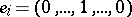,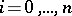(thestands in the-th place), i.e.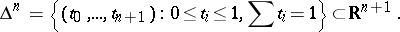For any topological space, the continuous mappings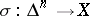are the singular simplices of(see Singular homology).

The simplicial complexwhose vertices are the points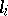,, while the simplices are arbitrary non-empty subsets of vertices. The geometric realization of this simplicial complex coincides with the standard simplex in the sense of 1).

The simplicial set, obtained by applying the functor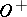to the simplicial scheme in 2), which is a contra-variant functor on the category(see Simplicial object in a category), for which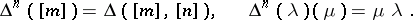Thus, non-decreasing sequences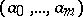of numbers fromare-dimensional simplices of the simplicial set, while the face operators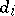and the degeneracy operators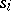of this simplicial set are defined by the formulas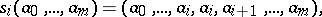where the sign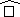signifies that the symbol beneath it is deleted. The simplicial set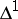is also called a simplicial segment. The simplex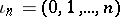(the unique non-degenerate-dimensional simplex of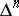) is called the fundamental simplex of. The smallest simplicial subset of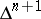containing all simplices of the form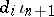with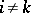is denoted by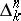and is called the-th standard horn.

For any simplicial setand an arbitrary-dimensional simplexof, there is a unique simplicial mappingfor which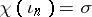. This mapping is said to be characteristic for.

The fundamental simplex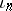of a simplicial set as in 3), which in this instance is denoted by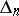.